# Machine Learning for Art

ml4a is a collection of tools and educational resources which apply techniques from machine learning to arts and creativity.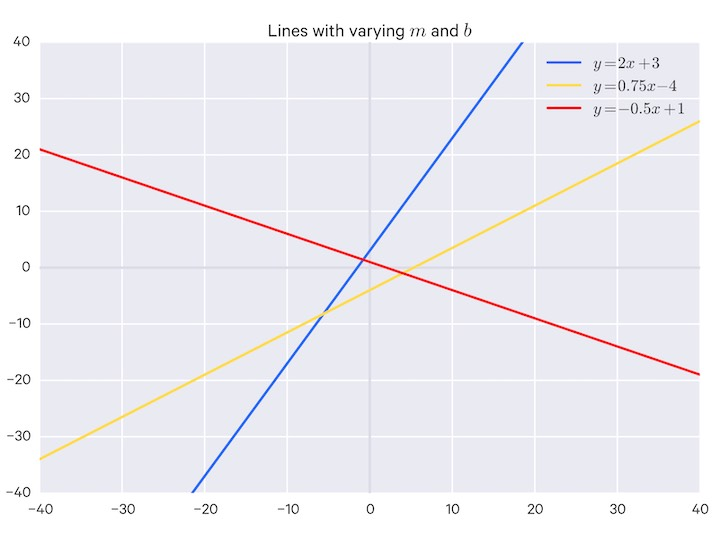##### Fundamentals of machine learning

Mathematical essentials for machine learning.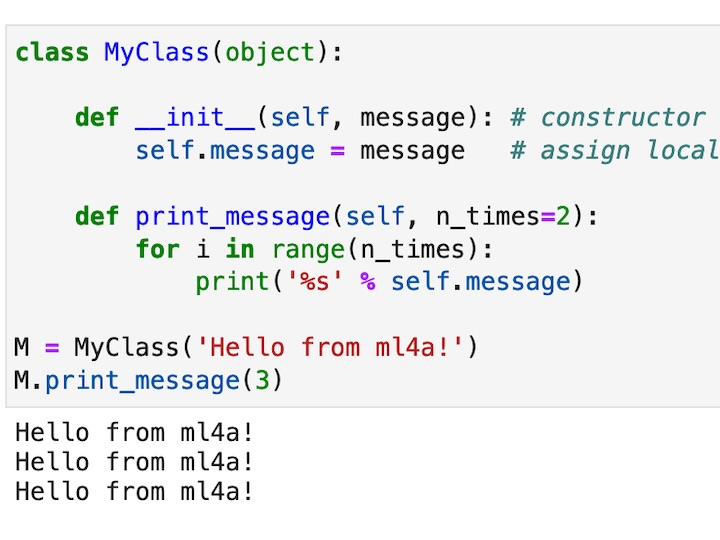##### Essentials of Python

A basic overview of programming with Python.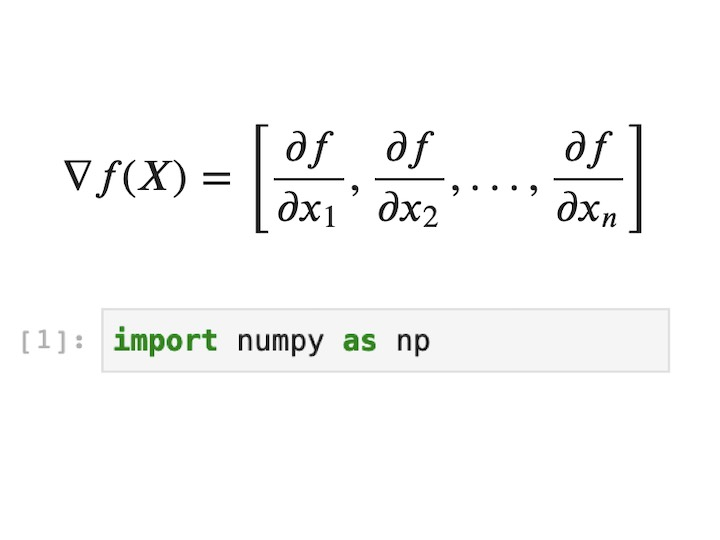##### Foundational mathematics

A review of relevant concepts from linear algebra and calculus, and intro to Numpy.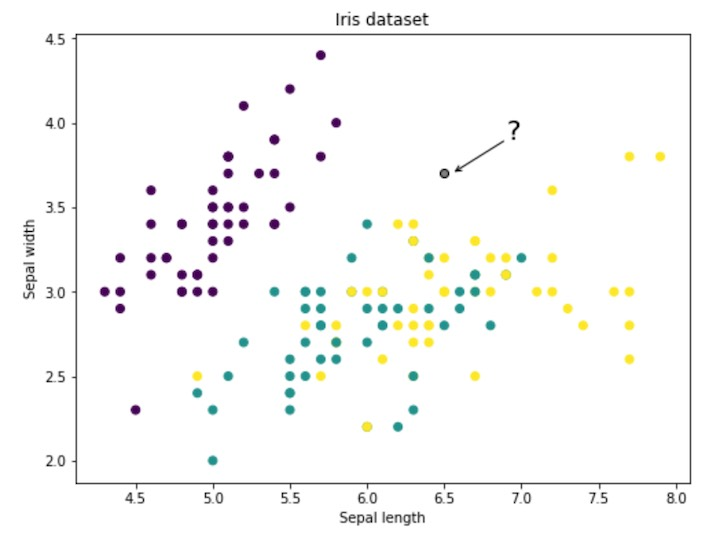##### Classification & k-Nearest Neighbors

Introduction to classification using k-nearest neighbors algorithm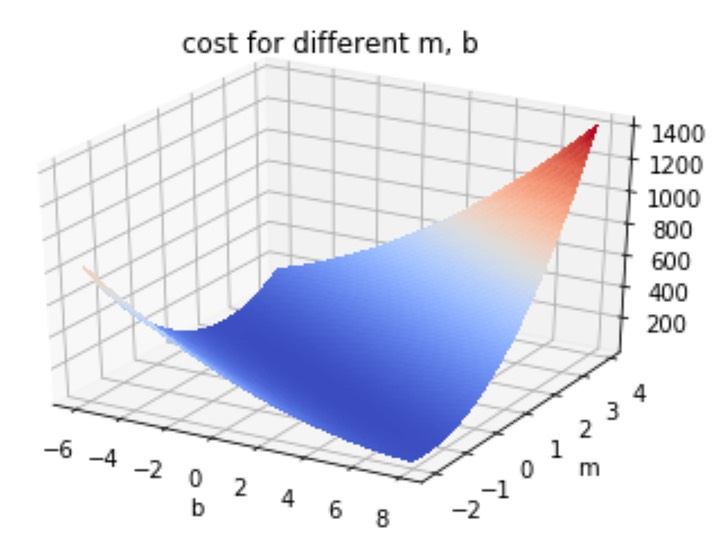##### Linear regression

Modeling data with line of best fit, basic gradient descent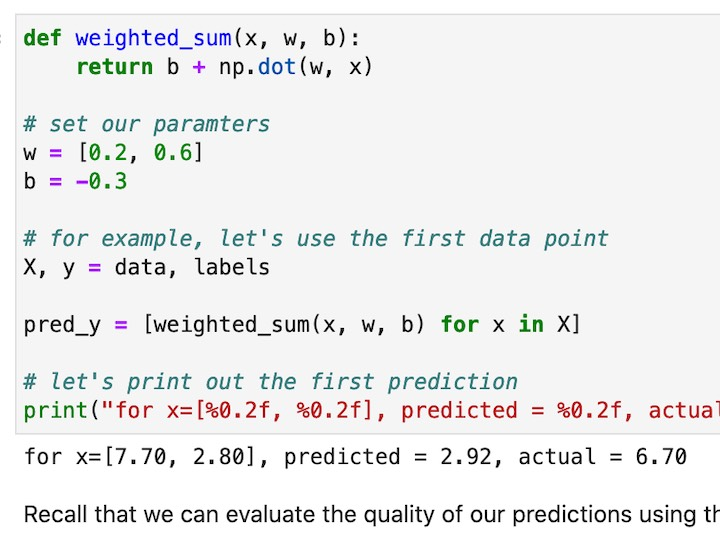##### DIY neural network

A numpy implementation of a neural network from scratch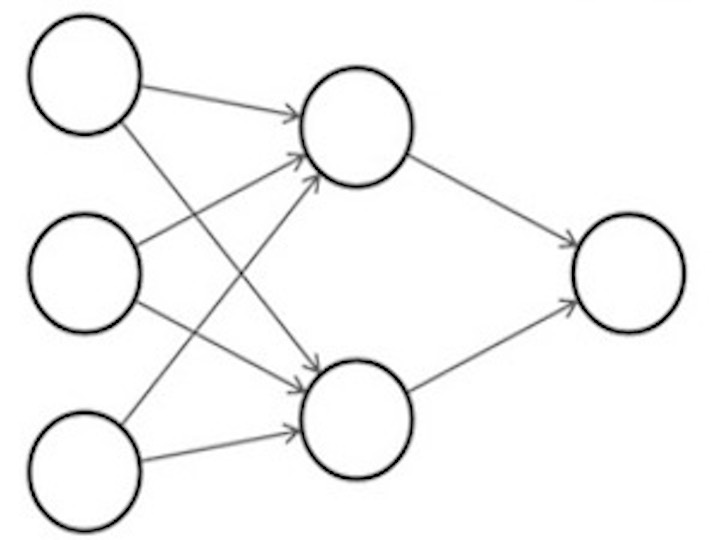##### Neural networks in Keras

How to train a simple feedforward neural network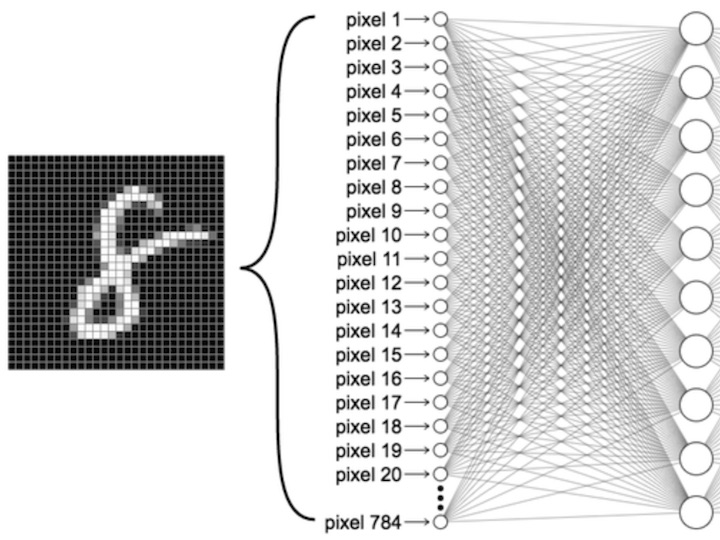##### Neural nets for classification

How to apply neural networks for classification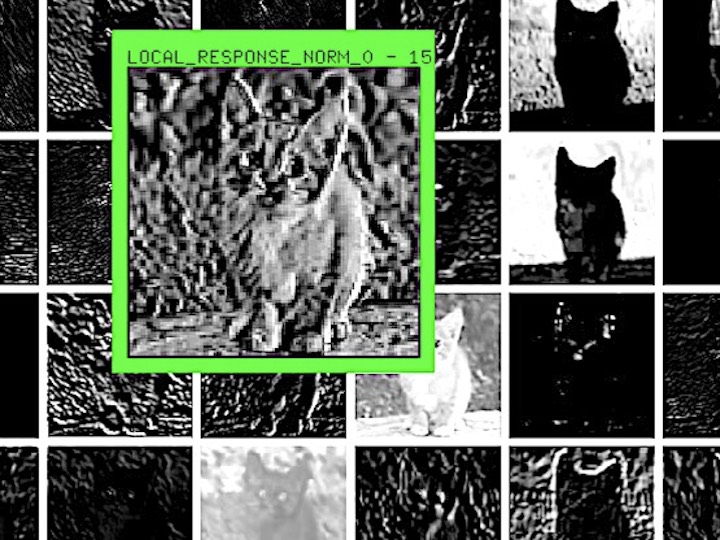##### Convolutional neural networks

How to train a convolutional neural network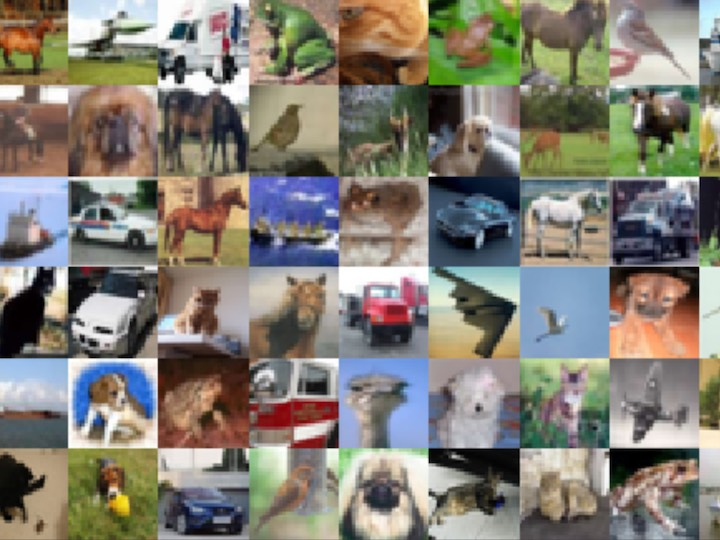##### Transfer learning

Training accurate image classifiers on small datasets